# removeobservable

Remove Sobol indices or elementary effects of observables

## Syntax

``results = removeobservable(gsaObj,obsNames)``

## Description

example

````results = removeobservable(gsaObj,obsNames)` removes the Sobol indices or elementary effects computed for the specified observables `obsNames` from `gsaResults`.```

## Examples

collapse all

`sbioloadproject tumor_growth_vpop_sa.sbproj`

Get a variant with the estimated parameters and the dose to apply to the model.

```v = getvariant(m1); d = getdose(m1,'interval_dose');```

Get the active configset and set the tumor weight as the response.

```cs = getconfigset(m1); cs.RuntimeOptions.StatesToLog = 'tumor_weight';```

Simulate the model and plot the tumor growth profile.

`sbioplot(sbiosimulate(m1,cs,v,d));`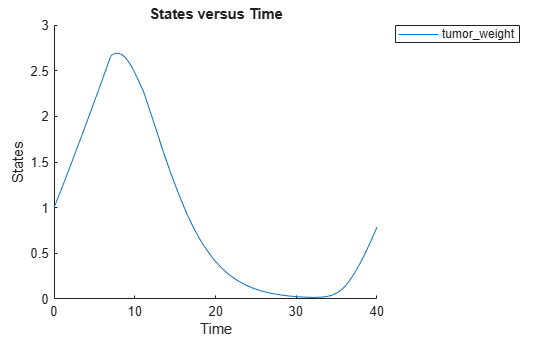Perform global sensitivity analysis (GSA) on the model to find the model parameters that the tumor growth is sensitive to.

First, retrieve model parameters of interest that are involved in the pharmacodynamics of the tumor growth. Define the model response as the tumor weight.

```modelParamNames = {'L0','L1','w0','k1','k2'}; outputName = 'tumor_weight';```

Then perform GSA by computing the first- and total-order Sobol indices using `sbiosobol`. Set `'ShowWaitBar'` to `true` to show the simulation progress. By default, the function uses 1000 parameter samples to compute the Sobol indices .

```rng('default'); sobolResults = sbiosobol(m1,modelParamNames,outputName,Variants=v,Doses=d,ShowWaitBar=true)```
```sobolResults = Sobol with properties: Time: [444x1 double] SobolIndices: [5x1 struct] Variance: [444x1 table] ParameterSamples: [1000x5 table] Observables: {'[Tumor Growth].tumor_weight'} SimulationInfo: [1x1 struct] ```

You can change the number of samples by specifying the `'NumberSamples'` name-value pair argument. The function requires a total of `(number of input parameters + 2) * NumberSamples` model simulations.

Show the mean model response, the simulation results, and a shaded region covering 90% of the simulation results.

`plotData(sobolResults,ShowMedian=true,ShowMean=false);`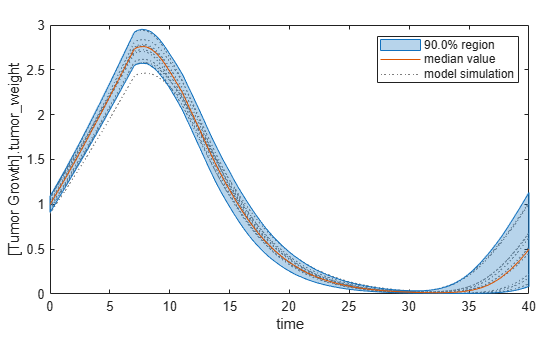You can adjust the quantile region to a different percentage by specifying `'Alphas' `for the lower and upper quantiles of all model responses. For instance, an alpha value of 0.1 plots a shaded region between the `100 * alpha` and `100 * (1 - alpha)` quantiles of all simulated model responses.

`plotData(sobolResults,Alphas=0.1,ShowMedian=true,ShowMean=false);`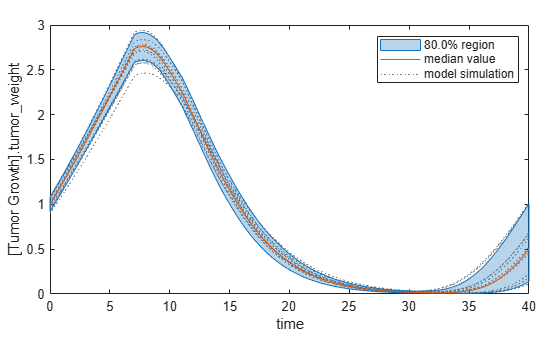Plot the time course of the first- and total-order Sobol indices.

```h = plot(sobolResults); % Resize the figure. h.Position(:) = [100 100 1280 800];```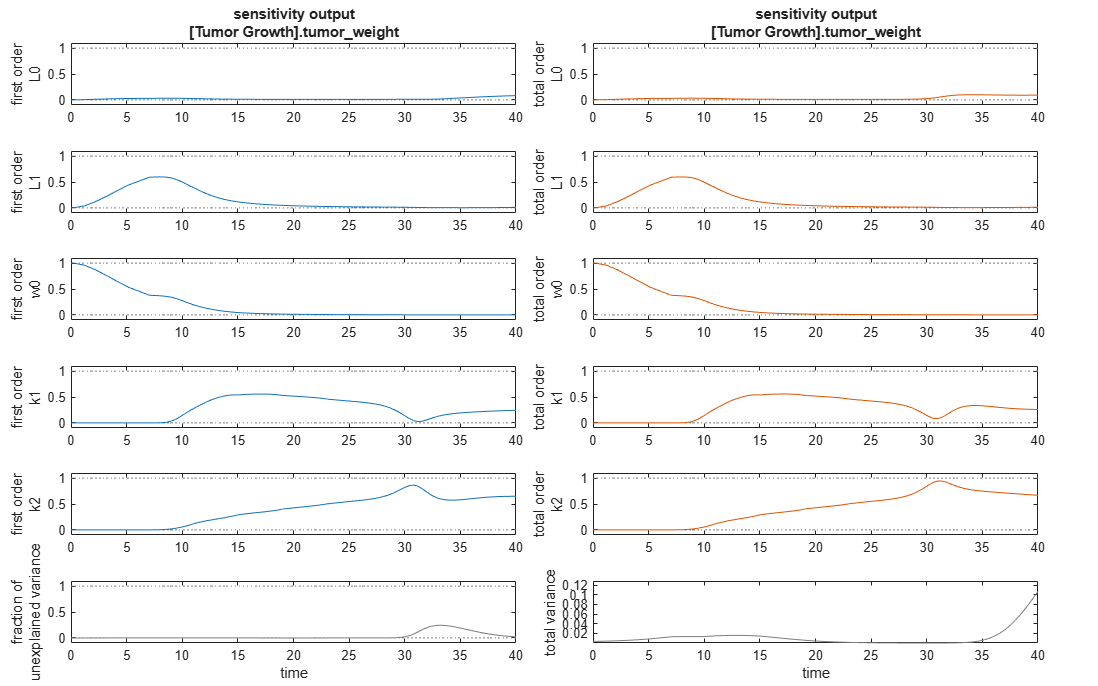The first-order Sobol index of an input parameter gives the fraction of the overall response variance that can be attributed to variations in the input parameter alone. The total-order index gives the fraction of the overall response variance that can be attributed to any joint parameter variations that include variations of the input parameter.

From the Sobol indices plots, parameters `L1` and `w0` seem to be the most sensitive parameters to the tumor weight before the dose was applied at t = 7. But after the dose is applied, `k1` and `k2` become more sensitive parameters and contribute most to the after-dosing stage of the tumor weight. The total variance plot also shows a larger variance for the after-dose stage at t > 35 than for the before-dose stage of the tumor growth, indicating that `k1` and `k2` might be more important parameters to investigate further. The fraction of unexplained variance shows some variance at around t = 33, but the total variance plot shows little variance at t = 33, meaning the unexplained variance could be insignificant. The fraction of unexplained variance is calculated as 1 - (sum of all the first-order Sobol indices), and the total variance is calculated using `var(response)`, where `response` is the model response at every time point.

You can also display the magnitudes of the sensitivities in a bar plot. Darker colors mean that those values occur more often over the whole time course.

`bar(sobolResults);`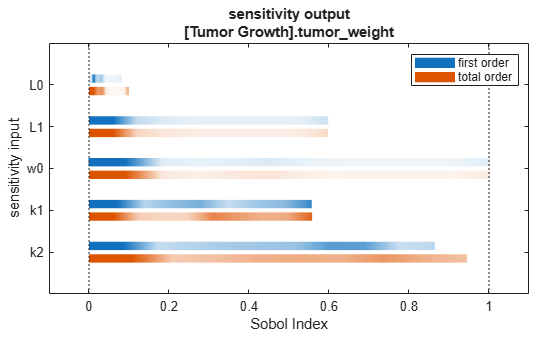You can specify more samples to increase the accuracy of the Sobol indices, but the simulation can take longer to finish. Use `addsamples` to add more samples. For example, if you specify 1500 samples, the function performs `1500 * (2 + number of input parameters)` simulations.

```gsaMoreSamples = addsamples(gsaResults,1500) ```

The SimulationInfo property of the result object contains various information for computing the Sobol indices. For instance, the model simulation data (SimData) for each simulation using a set of parameter samples is stored in the `SimData` field of the property. This field is an array of `SimData` objects.

`sobolResults.SimulationInfo.SimData`
``` SimBiology SimData Array : 1000-by-7 Index: Name: ModelName: DataCount: 1 - Tumor Growth Model 1 2 - Tumor Growth Model 1 3 - Tumor Growth Model 1 ... 7000 - Tumor Growth Model 1 ```

You can find out if any model simulation failed during the computation by checking the `ValidSample` field of `SimulationInfo`. In this example, the field shows no failed simulation runs.

`all(sobolResults.SimulationInfo.ValidSample)`
```ans = 1x7 logical array 1 1 1 1 1 1 1 ```

`SimulationInfo.ValidSample` is a table of logical values. It has the same size as `SimulationInfo.SimData`. If `ValidSample` indicates that any simulations failed, you can get more information about those simulation runs and the samples used for those runs by extracting information from the corresponding column of `SimulationInfo.SimDat`a. Suppose that the fourth column contains one or more failed simulation runs. Get the simulation data and sample values used for that simulation using `getSimulationResults`.

`[samplesUsed,sd,validruns] = getSimulationResults(sobolResults,4);`

You can add custom expressions as observables and compute Sobol indices for the added observables. For example, you can compute the Sobol indices for the maximum tumor weight by defining a custom expression as follows.

```% Suppress an information warning that is issued during simulation. warnSettings = warning('off', 'SimBiology:sbservices:SB_DIMANALYSISNOTDONE_MATLABFCN_UCON'); % Add the observable expression. sobolObs = addobservable(sobolResults,'Maximum tumor_weight','max(tumor_weight)','Units','gram');```

Plot the computed simulation results showing the 90% quantile region.

```h2 = plotData(sobolObs,ShowMedian=true,ShowMean=false); h2.Position(:) = [100 100 1280 800];```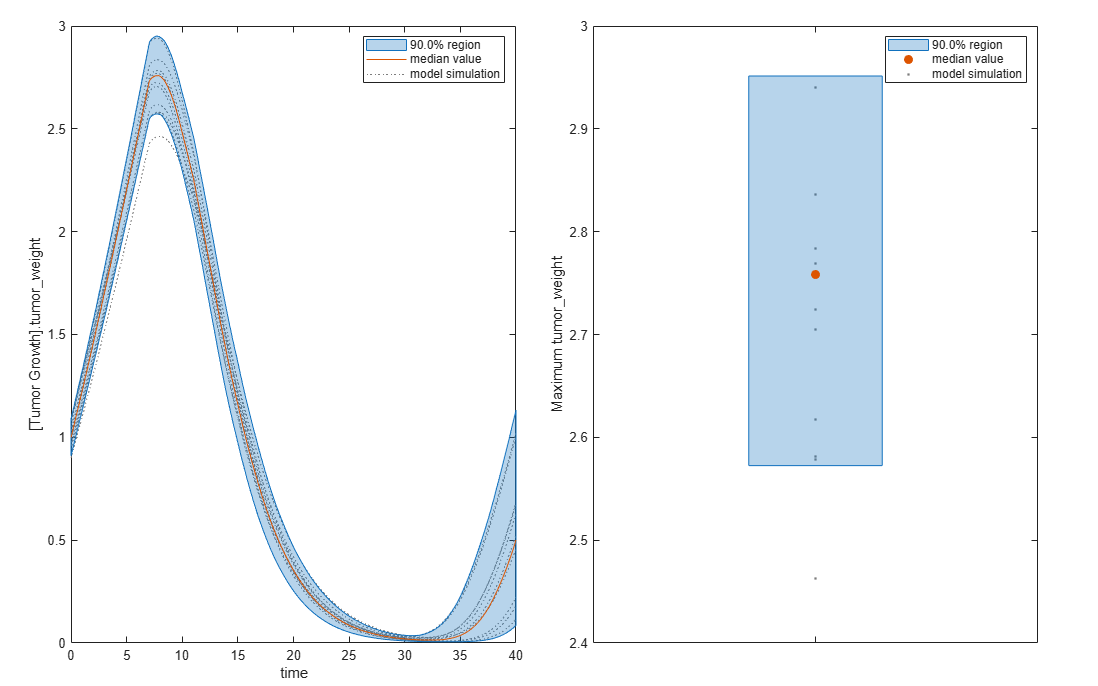You can also remove the observable by specifying its name.

`gsaNoObs = removeobservable(sobolObs,'Maximum tumor_weight');`

Restore the warning settings.

`warning(warnSettings);`

`sbioloadproject tumor_growth_vpop_sa.sbproj`

Get a variant with estimated parameters and the dose to apply to the model.

```v = getvariant(m1); d = getdose(m1,'interval_dose');```

Get the active configset and set the tumor weight as the response.

```cs = getconfigset(m1); cs.RuntimeOptions.StatesToLog = 'tumor_weight';```

Simulate the model and plot the tumor growth profile.

`sbioplot(sbiosimulate(m1,cs,v,d));`Perform global sensitivity analysis (GSA) on the model to find the model parameters that the tumor growth is sensitive to.

First, define model parameters of interest, which are involved in the pharmacodynamics of the tumor growth. Define the model response as the tumor weight.

```modelParamNames = {'L0','L1','w0','k1'}; outputName = 'tumor_weight';```

Then perform GSA by computing the elementary effects using `sbioelementaryeffects`. Use `100` as the number of samples and set `ShowWaitBar` to `true` to show the simulation progress.

```rng('default'); eeResults = sbioelementaryeffects(m1,modelParamNames,outputName,Variants=v,Doses=d,NumberSamples=100,ShowWaitbar=true);```

Show the median model response, the simulation results, and a shaded region covering 90% of the simulation results.

`plotData(eeResults,ShowMedian=true,ShowMean=false);`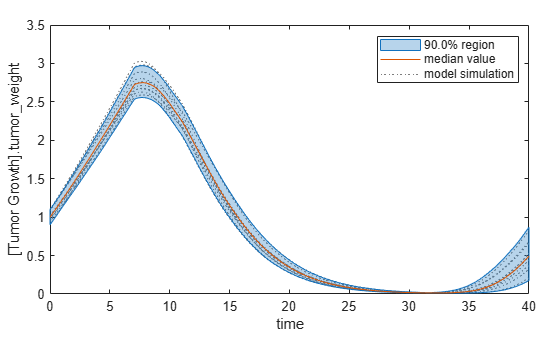You can adjust the quantile region to a different percentage by specifying `Alphas `for the lower and upper quantiles of all model responses. For instance, an `alpha` value of 0.1 plots a shaded region between the `100*alpha` and `100*(1-alpha)` quantiles of all simulated model responses.

`plotData(eeResults,Alphas=0.1,ShowMedian=true,ShowMean=false);`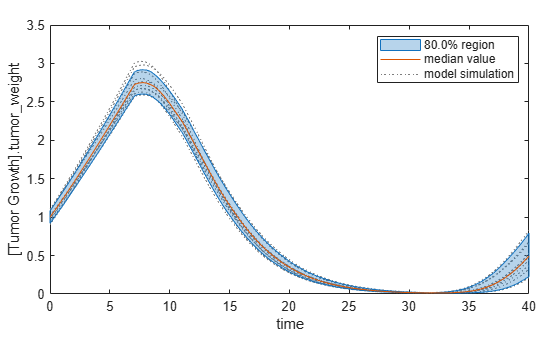Plot the time course of the means and standard deviations of the elementary effects.

```h = plot(eeResults); % Resize the figure. h.Position(:) = [100 100 1280 800];```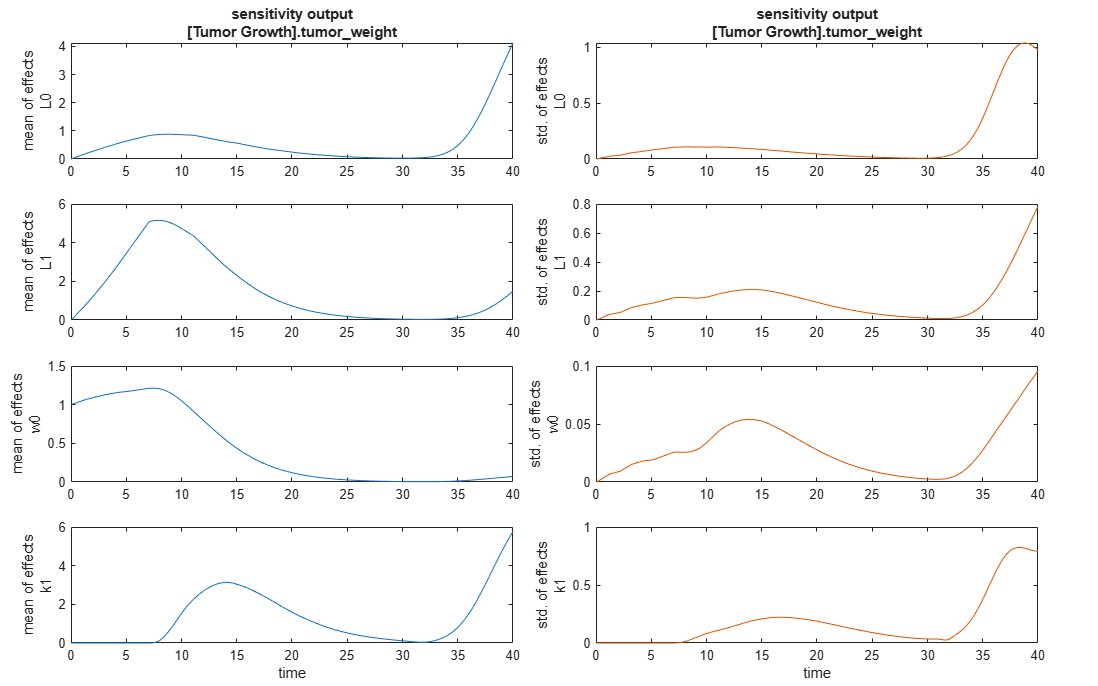The mean of effects explains whether variations in input parameter values have any effect on the tumor weight response. The standard deviation of effects explains whether the sensitivity change is dependent on the location in the parameter domain.

From the mean of effects plots, parameters `L1` and `w0` seem to be the most sensitive parameters to the tumor weight before the dose is applied at t = 7. But, after the dose is applied, `k1` and `L0` become more sensitive parameters and contribute most to the after-dosing stage of the tumor weight. The plots of standard deviation of effects show more deviations for the larger parameter values in the later stage (t > 35) than for the before-dose stage of the tumor growth.

You can also display the magnitudes of the sensitivities in a bar plot. Each color shading represents a histogram representing values at different times. Darker colors mean that those values occur more often over the whole time course.

`bar(eeResults);`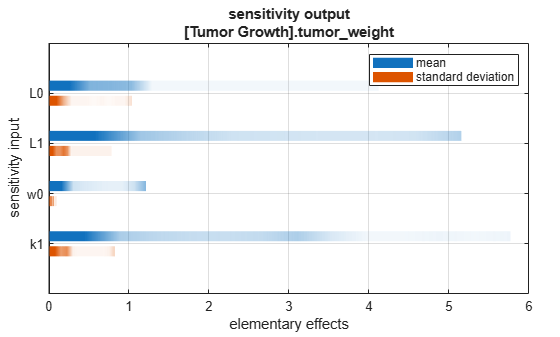You can also plot the parameter grids and samples used to compute the elementary effects.

`plotGrid(eeResults)`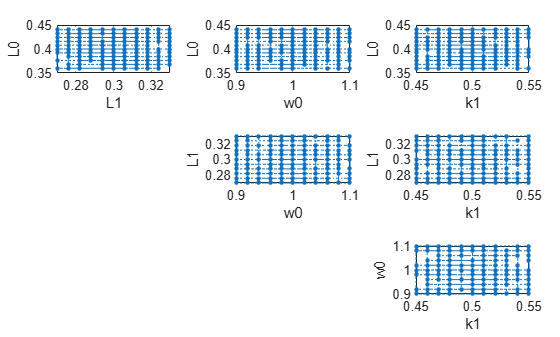You can specify more samples to increase the accuracy of the elementary effects, but the simulation can take longer to finish. Use `addsamples` to add more samples.

`eeResults2 = addsamples(eeResults,200);`

The `SimulationInfo` property of the result object contains various information for computing the elementary effects. For instance, the model simulation data (SimData) for each simulation using a set of parameter samples is stored in the `SimData` field of the property. This field is an array of `SimData` objects.

`eeResults2.SimulationInfo.SimData`
``` SimBiology SimData Array : 1500-by-1 Index: Name: ModelName: DataCount: 1 - Tumor Growth Model 1 2 - Tumor Growth Model 1 3 - Tumor Growth Model 1 ... 1500 - Tumor Growth Model 1 ```

You can find out if any model simulation failed during the computation by checking the `ValidSample` field of `SimulationInfo`. In this example, the field shows no failed simulation runs.

`all(eeResults2.SimulationInfo.ValidSample)`
```ans = logical 1 ```

You can add custom expressions as observables and compute the elementary effects of the added observables. For example, you can compute the effects for the maximum tumor weight by defining a custom expression as follows.

```% Suppress an information warning that is issued. warnSettings = warning('off', 'SimBiology:sbservices:SB_DIMANALYSISNOTDONE_MATLABFCN_UCON'); % Add the observable expression. eeObs = addobservable(eeResults2,'Maximum tumor_weight','max(tumor_weight)','Units','gram');```

Plot the computed simulation results showing the 90% quantile region.

```h2 = plotData(eeObs,ShowMedian=true,ShowMean=false); h2.Position(:) = [100 100 1500 800];```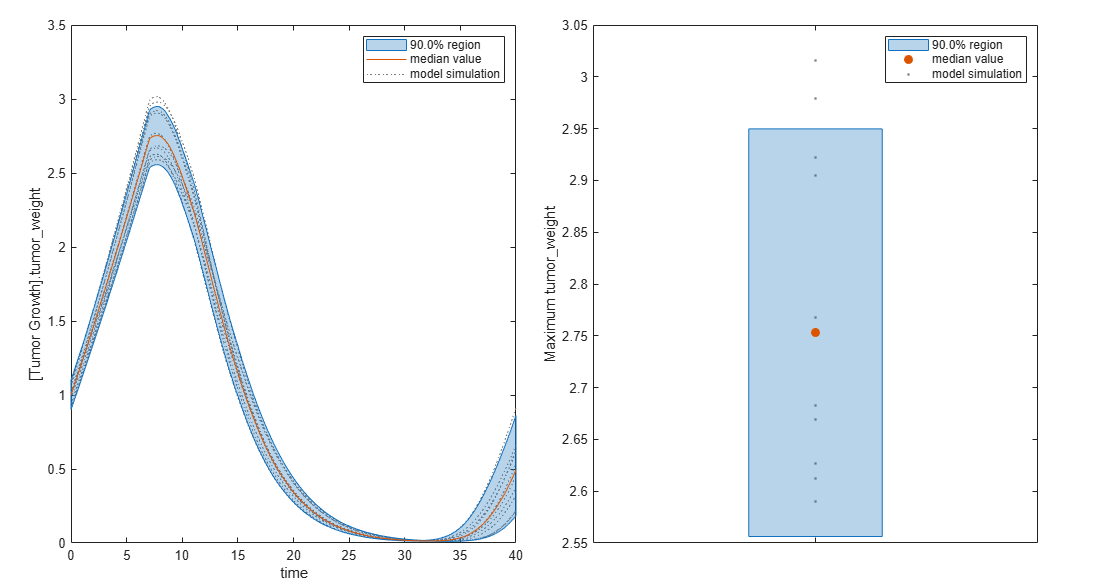You can also remove the observable by specifying its name.

`eeNoObs = removeobservable(eeObs,'Maximum tumor_weight');`

Restore the warning settings.

`warning(warnSettings);`

## Input Arguments

collapse all

Results from global sensitivity analysis, specified as a `SimBiology.gsa.Sobol` or `SimBiology.gsa.ElementaryEffects` object.

Names of observable expressions, specified as a character vector, string, string vector, or cell array of character vectors.

Data Types: `char` | `string` | `cell`

## Output Arguments

collapse all

Updated results after removal of the Sobol indices or elementary effects for specified observables, returned as a `SimBiology.gsa.Sobol` or `SimBiology.gsa.ElementaryEffects` object.

 Saltelli, Andrea, Paola Annoni, Ivano Azzini, Francesca Campolongo, Marco Ratto, and Stefano Tarantola. “Variance Based Sensitivity Analysis of Model Output. Design and Estimator for the Total Sensitivity Index.” Computer Physics Communications 181, no. 2 (February 2010): 259–70. https://doi.org/10.1016/j.cpc.2009.09.018.

 Morris, Max D. “Factorial Sampling Plans for Preliminary Computational Experiments.” Technometrics 33, no. 2 (May 1991): 161–74.

 Sohier, Henri, Jean-Loup Farges, and Helene Piet-Lahanier. “Improvement of the Representativity of the Morris Method for Air-Launch-to-Orbit Separation.” IFAC Proceedings Volumes 47, no. 3 (2014): 7954–59.